|

# 出租房大变身!井井有条的北欧风无限治愈强迫症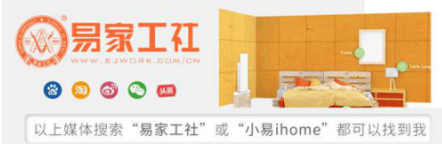海量装修设计精品案例来自厦门市十佳装饰设计企业——易家工社

房屋位置：泉水湾（厦门集美）

房屋户型：三房一卫94㎡

风格：北欧风格

装修公司：易家工社

设计师：罗奕贤

L先生（业主）入手的这套房子位于集美区泉水湾，前身是出租房，环境老化破旧，动线杂乱。所以就迫不及待的把装修提上日程，希望在年底前（2018年）住上新家。

迄今已经入住了半年多了，听说我们最近有在做家访，便发了消息给设计师再次邀请我们到家中做客。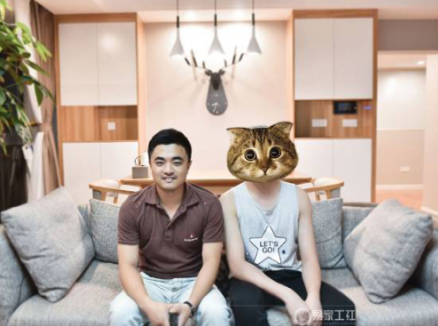▲设计师罗奕贤（左）和L先生（右）

为了赶工期，量房的时候，房子里还住着其他租客，设计师和助理在杂乱的物品和满地的排插线中小心翼翼穿梭着。L先生说：朋友们都曾劝阻过我找装修公司，说是后期增项太多，但最后我还是找了，一是为了可以更快的入住，二是在对比了之后发现还是有可以信任的装修公司的。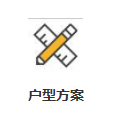L先生是工程科班出身，做事严谨且井井有条，有轻微的强迫症。毕业以后一直过着熬夜赶图赶工的日子，淹没在鹭岛匆匆人流之中。他说：曾经说好的诗与远方，也在不知不觉中渐行渐远。

所以设计师不仅仅要满足L先生的严格要求，更是要打造一个可寄托疲惫身心的乌托邦。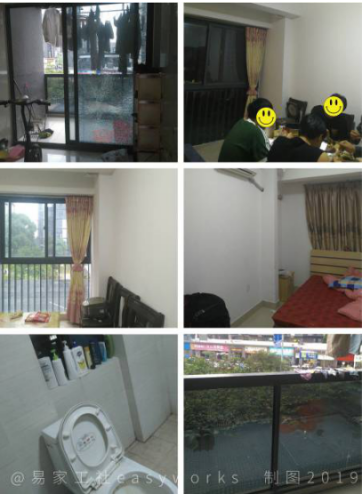▲改造前：租房一族熟悉的场景

本户型的原始结构并没有做多大的改动，94㎡的空间对于现阶段的两口之家来说十分充裕，也可以满足以后的成长性家庭需求，三个房间住下三代同堂一点问题没有。

比较多的问题就是原来的内部裸露管道实在太多，公卫门也直直冲着入户门，这对于L先生来说简直是无法接受的。同时他也希望整个空间的界限更明显，收纳空间更多一些。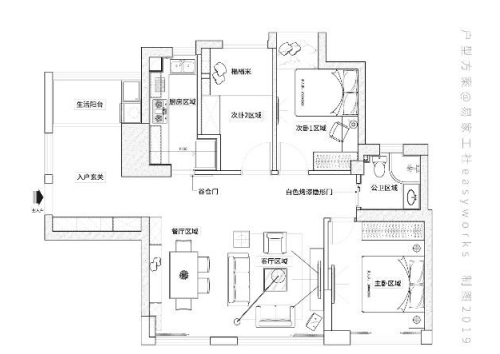▲户型方案：修改以后的空间布置

● 阳台与餐厅下水管用衣柜巧妙隐藏

● 公卫门对着入户，做了一个隐形门

● 厨房从榻榻米房借空间，腾出冰箱的位置

● 次卧门的位置更改：腾出衣柜区，避免床头靠窗

● 餐厅的空间太窄，卡座和餐边柜充分利用空间

整个方案规划下来显得更井井有条，严谨。也解决了原来的小问题。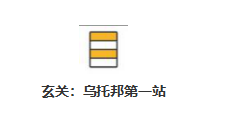一进门的玄关非常宽敞，除了右侧的鞋柜区，左边有一大块开阔地与生活阳台相连。一开始设计师和梁先生探讨砌个墙把这块区域单独利用起来，后来这个想法遭到了推翻。

L先生说他更喜欢一进门就是开阔什么都没有的感觉，那样心情才觉得舒畅。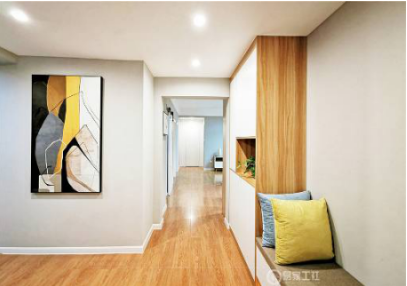▲进门视角

地板是和客餐厅一样木纹瓷砖（全屋基本都是这个调调），按照设计师的搭配，右半边做了到顶的玄关柜，另一半是一个带有长椅的鞋柜，边上放着几个抱枕，平时回家或出门在这里坐着小憩一下也是不错的选择。

一开始L先生准备在玄关顶部安装感应灯，后来觉得可能太显眼了便把灯藏到了鞋柜底下，即不打扰也十分美观。

柜子和抽屉全都做了无拉手的设计，也符合L先生的喜好，看起来非常简洁规范。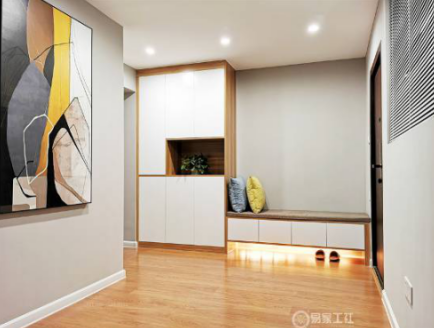▲玄关柜，鞋柜区

左边的空间除了生活阳台什么都没有，以后可能会添加一些健身设备，所以预留了插座。

浅灰色的墙空荡荡的太突兀，便挂上了一副不错的油画点缀。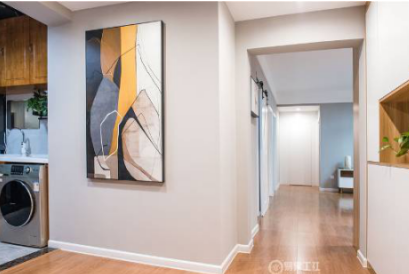▲生活阳台

生活阳台保留了原来出租房的阳光吊顶，改造完的玻璃窗，增加了低层楼房的安全性，白天阳光可以直射进来，自动晾晒架自带的照明晒衣服很方便。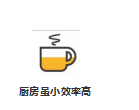厨房的空间很小，橱柜另一边只能放一个洗菜池，原来的冰箱都是放在客厅，执念于功能区域界线观念的L先生，让设计师从隔壁借了“一亩三分地”把冰箱放了进去。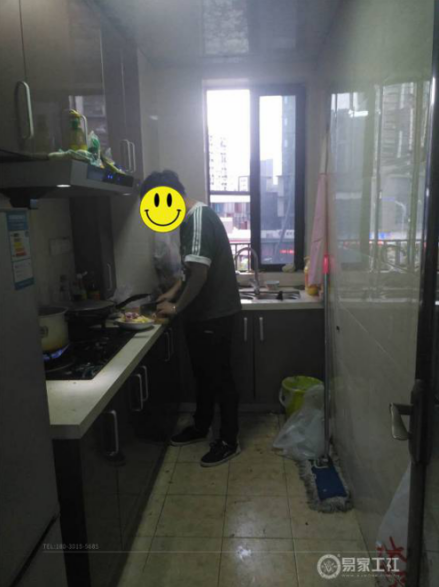▲厨房改造前

入口做了谷仓门，橱柜依然是无拉手设计。

地柜暗有玄机，隐藏着前置净水器、小厨宝、消毒柜等设备。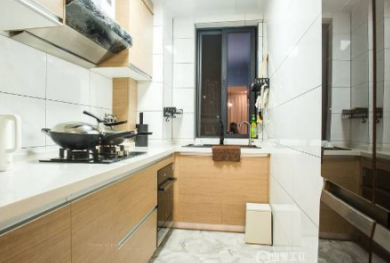▲厨房改造前后对比

由于L先生家庭的工作原因，平时并没有经常在家中一日三餐，不过为了以后家庭成长性需求，该有的功能都一次性准备好了，唯一没有改变的还是一如既往的整洁干净，井井有条。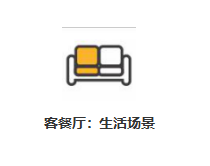客餐厅：生活场景

L先生想把走廊处的视野保留，于是客餐厅的空间就无法延伸出来，就显得紧凑了一些。由于原来的出租屋的缺陷，角落的下水管裸露在外面，简直逼死强迫症。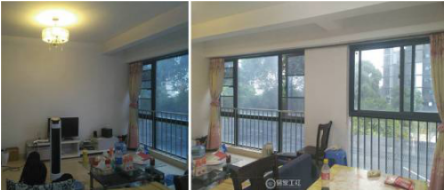▲客餐厅改造前

全房的墙壁用的都是水性硅藻泥，在南方空气湿度比较高的地方特别是低层楼房，做“会呼吸”的硅藻泥还是一个不错的选择，最好是做全房。

L先生一开始在色卡上选择的是比较深的灰色，在设计师的建议下改成了比较百搭的浅灰色，最后呈现出的效果也比较干净简洁。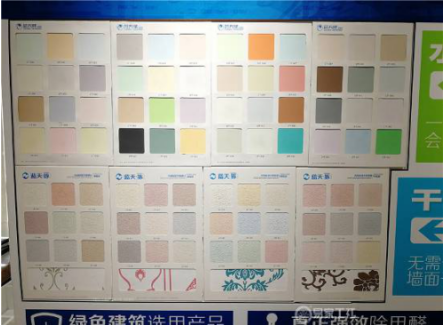最后选择的是013色号。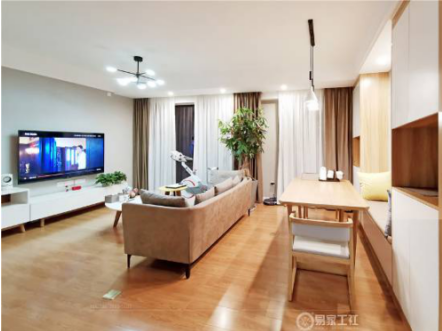▲客餐厅改造后

沙发旁边放着的健身器材和招财树，落地窗的阳光很好，有绿植可以优化一下室内的空气，还有一个不起眼的扫地机器人，L先生说这个非常实用。

家具也都是北欧风高脚小细腿，方便扫地机器人干活。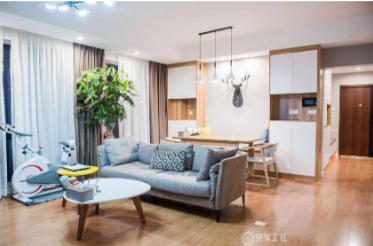▲客厅纵深

L先生的话就不考虑大型沙发了，选择了小巧的三座沙发放在中间，版型也是比较直挺挺的，不怎么占用空间，虽然坐上会感觉有点硬，没有松垮的感觉。但他非常喜欢这个沙发，电脑放到边几上，在这里做工作的话，这样的沙发也容易打起精神来，颜值也很高。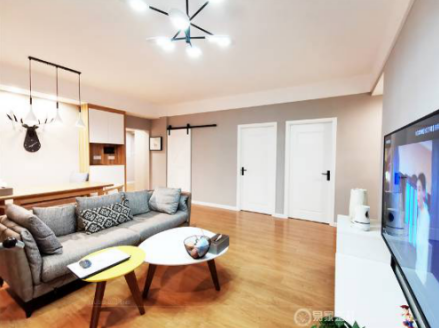▲休闲工作的天地

就是有一点不太好，脚总是不自觉的伸到前面的茶几上，说完这句话L先生偷瞄了一眼正在吃苹果的设计师。

电视柜是白色烤瓷质感+桃木纹底座，和定制柜体非常搭。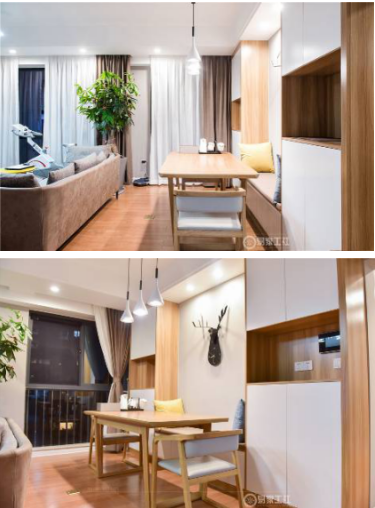▲餐厅卡座区

餐厅由于纵深紧凑的原因，选用了卡座的形式，平时两个人吃饭喝茶非常的实用。餐边柜不仅仅可以收纳各种杂乱物品，还巧妙的遮挡了之前裸露的下水管，无拉手设计的定制柜配上浅灰色墙体好看极了。

北欧风的简约灯具3个灯泡为6w，色温3000K，柔和的刚刚好，打在纯实木的餐桌上堪比饭店精修图。

非常治愈且丰富的生活场景。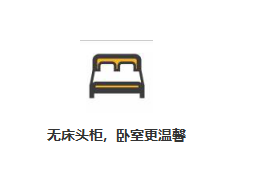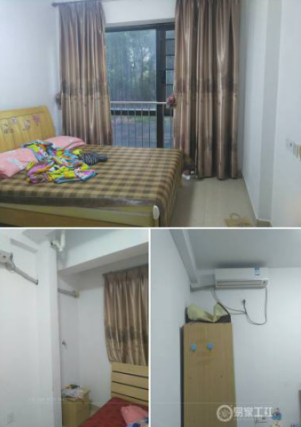▲卧室改造前

主卧在户型的最角落，也是最安静的地方。满墙的定制衣柜，黑白的配色，无拉手设计显得更加的高大上，几乎可以收纳下所有的衣物了。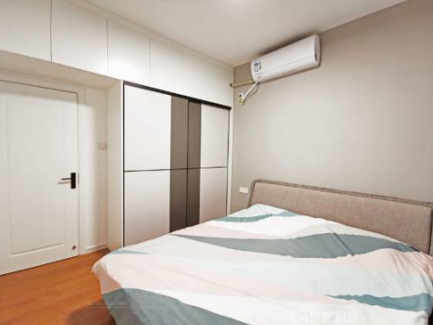▲主卧：配色舒服

原来的方案设计了床头柜，后来因为觉得把床头柜去掉不仅仅比较宽敞些，空间布局规划的很明确，也更加显得井井有条了。L先生说，位于低层其实好处也挺多的，与小区的树木齐高，每天早上都能看到小区里的不知名小鸟站在窗后，影子映在薄纱窗帘上，非常有意境。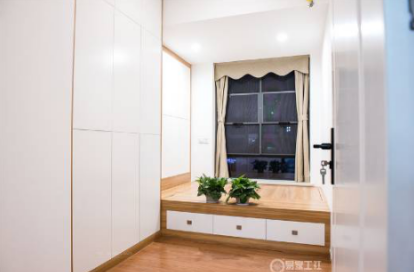▲无床头柜

次卧的格局和主卧基本一致，门的位置做了移动，这样就腾出了衣柜的位置。

其实衣柜就像积木一样，可以根据房间大小和自己的衣服多少，组成不同形状不同的高度，配合各种裤夹、抽屉，衣架，特别灵活方便。

▲榻榻米房

厨房和次卧之间就是榻榻米房，目前都处于闲置状态，墙漆还原到白色，榻榻米床衣柜与客厅玄关是一种款式。收纳空间比主卧还要多上一些，有些大件的棉被什么的都被挪到了这里。

包容性很强，日后可根据家庭的成长性自由布置，百搭使用。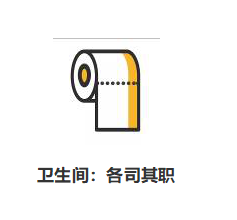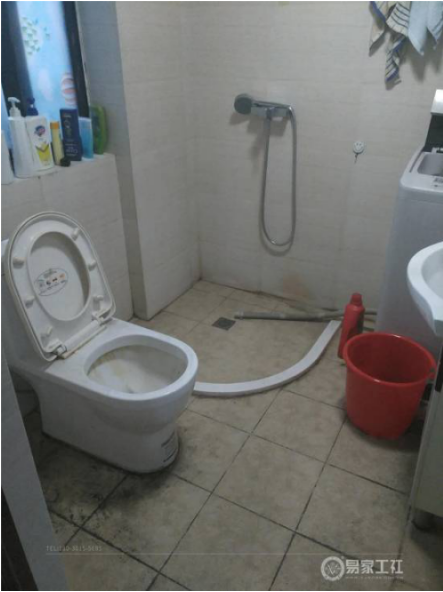▲卫生间改造前

由于卫生间的门对着入户，长长的走道一览无余，于是便将卫生间的门做成了白色烤漆隐形门，从玄关看过来基本看不到痕迹，整洁美观。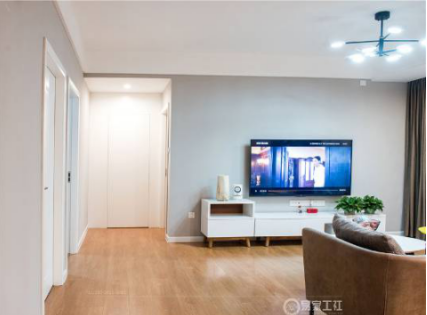▲卫生间隐形门

仅有3㎡的空间分置于三个角落，马桶和洗手池隔开，淋浴房做个中间者，裸露的下水管做了包管，打扫很方便。洗脸，如厕，洗澡基本的生活功能都能满足。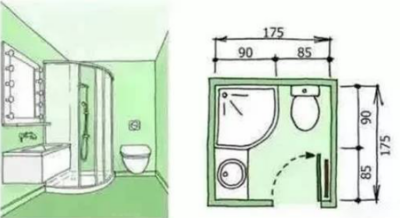唯一的缺点就是，没有多余的空间可以收纳物品了。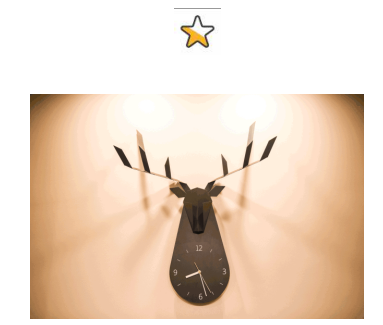装修开始到入住半年，L先生对于这个家的期望在一步步的实现之中，虽然施工中也遇到了一些麻烦和遗憾，但当自己参与到家慢慢成型过程中的时候，会有更多的感悟大于遗憾。

按照他的话来说就是，能让我这个严格的强迫症看起来舒服，就很OK。装修也不是随心所欲的改造。

是的，再次到访，我们也真真切切感受到了L先生对这个家的喜爱和期待。

`声明：本文由入驻焦点开放平台的作者撰写，除焦点官方账号外，观点仅代表作者本人，不代表焦点立场错误信息举报电话： 400-099-0099，邮箱：jubao@vip.sohu.com，或点此进行意见反馈，或点此进行举报投诉。`A B C D E F G H J K L M N P Q R S T W X Y Z
A - B - C - D - E
• A
• 鞍山
• 安庆
• 安阳
• 安顺
• 安康
• 澳门
• B
• 北京
• 保定
• 包头
• 巴彦淖尔
• 本溪
• 蚌埠
• 亳州
• 滨州
• 北海
• 百色
• 巴中
• 毕节
• 保山
• 宝鸡
• 白银
• 巴州
• C
• 承德
• 沧州
• 长治
• 赤峰
• 朝阳
• 长春
• 常州
• 滁州
• 池州
• 长沙
• 常德
• 郴州
• 潮州
• 崇左
• 重庆
• 成都
• 楚雄
• 昌都
• 慈溪
• 常熟
• D
• 大同
• 大连
• 丹东
• 大庆
• 东营
• 德州
• 东莞
• 德阳
• 达州
• 大理
• 德宏
• 定西
• 儋州
• 东平
• E
• 鄂尔多斯
• 鄂州
• 恩施
F - G - H - I - J
• F
• 抚顺
• 阜新
• 阜阳
• 福州
• 抚州
• 佛山
• 防城港
• G
• 赣州
• 广州
• 桂林
• 贵港
• 广元
• 广安
• 贵阳
• 固原
• H
• 邯郸
• 衡水
• 呼和浩特
• 呼伦贝尔
• 葫芦岛
• 哈尔滨
• 黑河
• 淮安
• 杭州
• 湖州
• 合肥
• 淮南
• 淮北
• 黄山
• 菏泽
• 鹤壁
• 黄石
• 黄冈
• 衡阳
• 怀化
• 惠州
• 河源
• 贺州
• 河池
• 海口
• 红河
• 汉中
• 海东
• I
• J
• 晋中
• 锦州
• 吉林
• 鸡西
• 佳木斯
• 嘉兴
• 金华
• 景德镇
• 九江
• 吉安
• 济南
• 济宁
• 焦作
• 荆门
• 荆州
• 江门
• 揭阳
• 金昌
• 酒泉
• 嘉峪关
K - L - M - N - P
• K
• 开封
• 昆明
• 昆山
• L
• 廊坊
• 临汾
• 辽阳
• 连云港
• 丽水
• 六安
• 龙岩
• 莱芜
• 临沂
• 聊城
• 洛阳
• 漯河
• 娄底
• 柳州
• 来宾
• 泸州
• 乐山
• 六盘水
• 丽江
• 临沧
• 拉萨
• 林芝
• 兰州
• 陇南
• M
• 牡丹江
• 马鞍山
• 茂名
• 梅州
• 绵阳
• 眉山
• N
• 南京
• 南通
• 宁波
• 南平
• 宁德
• 南昌
• 南阳
• 南宁
• 内江
• 南充
• P
• 盘锦
• 莆田
• 平顶山
• 濮阳
• 攀枝花
• 普洱
• 平凉
Q - R - S - T - W
• Q
• 秦皇岛
• 齐齐哈尔
• 衢州
• 泉州
• 青岛
• 清远
• 钦州
• 黔南
• 曲靖
• 庆阳
• R
• 日照
• 日喀则
• S
• 石家庄
• 沈阳
• 双鸭山
• 绥化
• 上海
• 苏州
• 宿迁
• 绍兴
• 宿州
• 三明
• 上饶
• 三门峡
• 商丘
• 十堰
• 随州
• 邵阳
• 韶关
• 深圳
• 汕头
• 汕尾
• 三亚
• 三沙
• 遂宁
• 山南
• 商洛
• 石嘴山
• T
• 天津
• 唐山
• 太原
• 通辽
• 铁岭
• 泰州
• 台州
• 铜陵
• 泰安
• 铜仁
• 铜川
• 天水
• 天门
• W
• 乌海
• 乌兰察布
• 无锡
• 温州
• 芜湖
• 潍坊
• 威海
• 武汉
• 梧州
• 渭南
• 武威
• 吴忠
• 乌鲁木齐
X - Y - Z
• X
• 邢台
• 徐州
• 宣城
• 厦门
• 新乡
• 许昌
• 信阳
• 襄阳
• 孝感
• 咸宁
• 湘潭
• 湘西
• 西双版纳
• 西安
• 咸阳
• 西宁
• 仙桃
• 西昌
• Y
• 运城
• 营口
• 盐城
• 扬州
• 鹰潭
• 宜春
• 烟台
• 宜昌
• 岳阳
• 益阳
• 永州
• 阳江
• 云浮
• 玉林
• 宜宾
• 雅安
• 玉溪
• 延安
• 榆林
• 银川
• Z
• 张家口
• 镇江
• 舟山
• 漳州
• 淄博
• 枣庄
• 郑州
• 周口
• 驻马店
• 株洲
• 张家界
• 珠海
• 湛江
• 肇庆
• 中山
• 自贡
• 资阳
• 遵义
• 昭通
• 张掖
• 中卫

1室1厅1厨1卫1阳台

1
2
3
4
5

0
1
2

1

1

0
1
2
3报名成功，资料已提交审核A B C D E F G H J K L M N P Q R S T W X Y Z
A - B - C - D - E
• A
• 鞍山
• 安庆
• 安阳
• 安顺
• 安康
• 澳门
• B
• 北京
• 保定
• 包头
• 巴彦淖尔
• 本溪
• 蚌埠
• 亳州
• 滨州
• 北海
• 百色
• 巴中
• 毕节
• 保山
• 宝鸡
• 白银
• 巴州
• C
• 承德
• 沧州
• 长治
• 赤峰
• 朝阳
• 长春
• 常州
• 滁州
• 池州
• 长沙
• 常德
• 郴州
• 潮州
• 崇左
• 重庆
• 成都
• 楚雄
• 昌都
• 慈溪
• 常熟
• D
• 大同
• 大连
• 丹东
• 大庆
• 东营
• 德州
• 东莞
• 德阳
• 达州
• 大理
• 德宏
• 定西
• 儋州
• 东平
• E
• 鄂尔多斯
• 鄂州
• 恩施
F - G - H - I - J
• F
• 抚顺
• 阜新
• 阜阳
• 福州
• 抚州
• 佛山
• 防城港
• G
• 赣州
• 广州
• 桂林
• 贵港
• 广元
• 广安
• 贵阳
• 固原
• H
• 邯郸
• 衡水
• 呼和浩特
• 呼伦贝尔
• 葫芦岛
• 哈尔滨
• 黑河
• 淮安
• 杭州
• 湖州
• 合肥
• 淮南
• 淮北
• 黄山
• 菏泽
• 鹤壁
• 黄石
• 黄冈
• 衡阳
• 怀化
• 惠州
• 河源
• 贺州
• 河池
• 海口
• 红河
• 汉中
• 海东
• I
• J
• 晋中
• 锦州
• 吉林
• 鸡西
• 佳木斯
• 嘉兴
• 金华
• 景德镇
• 九江
• 吉安
• 济南
• 济宁
• 焦作
• 荆门
• 荆州
• 江门
• 揭阳
• 金昌
• 酒泉
• 嘉峪关
K - L - M - N - P
• K
• 开封
• 昆明
• 昆山
• L
• 廊坊
• 临汾
• 辽阳
• 连云港
• 丽水
• 六安
• 龙岩
• 莱芜
• 临沂
• 聊城
• 洛阳
• 漯河
• 娄底
• 柳州
• 来宾
• 泸州
• 乐山
• 六盘水
• 丽江
• 临沧
• 拉萨
• 林芝
• 兰州
• 陇南
• M
• 牡丹江
• 马鞍山
• 茂名
• 梅州
• 绵阳
• 眉山
• N
• 南京
• 南通
• 宁波
• 南平
• 宁德
• 南昌
• 南阳
• 南宁
• 内江
• 南充
• P
• 盘锦
• 莆田
• 平顶山
• 濮阳
• 攀枝花
• 普洱
• 平凉
Q - R - S - T - W
• Q
• 秦皇岛
• 齐齐哈尔
• 衢州
• 泉州
• 青岛
• 清远
• 钦州
• 黔南
• 曲靖
• 庆阳
• R
• 日照
• 日喀则
• S
• 石家庄
• 沈阳
• 双鸭山
• 绥化
• 上海
• 苏州
• 宿迁
• 绍兴
• 宿州
• 三明
• 上饶
• 三门峡
• 商丘
• 十堰
• 随州
• 邵阳
• 韶关
• 深圳
• 汕头
• 汕尾
• 三亚
• 三沙
• 遂宁
• 山南
• 商洛
• 石嘴山
• T
• 天津
• 唐山
• 太原
• 通辽
• 铁岭
• 泰州
• 台州
• 铜陵
• 泰安
• 铜仁
• 铜川
• 天水
• 天门
• W
• 乌海
• 乌兰察布
• 无锡
• 温州
• 芜湖
• 潍坊
• 威海
• 武汉
• 梧州
• 渭南
• 武威
• 吴忠
• 乌鲁木齐
X - Y - Z
• X
• 邢台
• 徐州
• 宣城
• 厦门
• 新乡
• 许昌
• 信阳
• 襄阳
• 孝感
• 咸宁
• 湘潭
• 湘西
• 西双版纳
• 西安
• 咸阳
• 西宁
• 仙桃
• 西昌
• Y
• 运城
• 营口
• 盐城
• 扬州
• 鹰潭
• 宜春
• 烟台
• 宜昌
• 岳阳
• 益阳
• 永州
• 阳江
• 云浮
• 玉林
• 宜宾
• 雅安
• 玉溪
• 延安
• 榆林
• 银川
• Z
• 张家口
• 镇江
• 舟山
• 漳州
• 淄博
• 枣庄
• 郑州
• 周口
• 驻马店
• 株洲
• 张家界
• 珠海
• 湛江
• 肇庆
• 中山
• 自贡
• 资阳
• 遵义
• 昭通
• 张掖
• 中卫• 手机• 分享
• 设计
免费设计
• 计算器
装修计算器
• 入驻
合作入驻
• 联系
联系我们
• 置顶
返回顶部